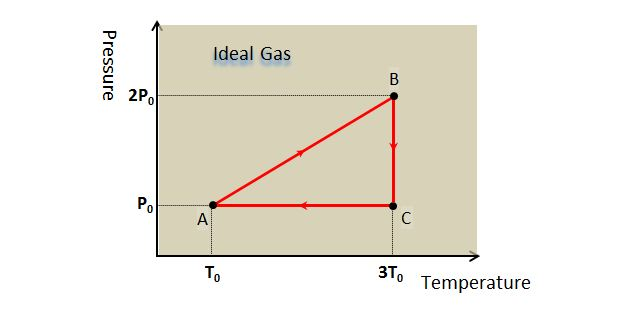# Volume change of ideal gasThe above is the pressure-temperature diagram of a given mass of ideal gas which is circulating by the sequence A $\to$ B $\to$ C $\to$ A. Let V$_A$, V$_B$ and V$_C$ denote the volumes at A, B and C, respectively, then what is the ratio $V_A:V_B:V_C?$

×

Problem Loading...

Note Loading...

Set Loading...